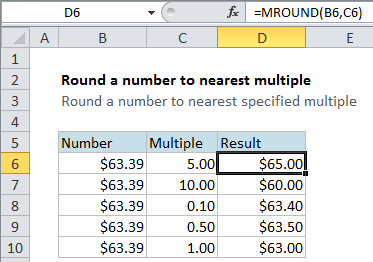Round a number to nearest multiple in Excel

This tutorials shows how to Round a number to nearest multiple in Excel.

If you need to round a number to the nearest specified multiple (i.e. round a number to the nearest dollar, nearest \$.25, nearest multiple of 5 or 10, etc) you can use the MROUND function.

Formula

`=MROUND(number,multiple)`Explanation

In the example, the formula in cell D6 is

`=MROUND(B6,C6)`

This tells Excel to take the value in B6 (\$63.39 ) and round it to the nearest multiple of the value in C6 (5). The result is \$65.00, since 65 is the nearest multiple of 5 to 63.39.

Also See:   Round time to nearest 15 minutes in Excel

You can use MROUND to round prices, times, instrument readings or any other numeric value.

Note that MROUND always rounds to the nearest value using the specified multiple. If you need to round either up or down using a multiple, use the CEILING or FLOOR functions.